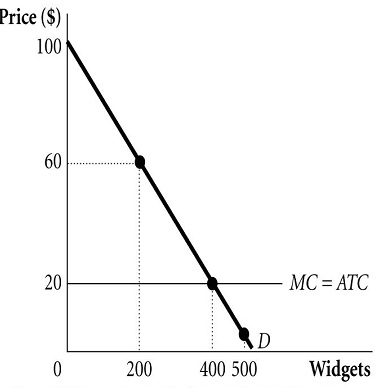# Refer to the figure below to answer the questions that follow. a. Suppose a monopolist faces the...

## Question:

Refer to the figure below to answer the questions that follow.a. Suppose a monopolist faces the demand and costs in the figure above and is able to perfectly price discriminate. How many widgets does the monopoly supply when they act so as to maximize their profits?

How much profit does the monopolist earn?

b. If the monopolist is able to perfectly price discriminate. How much surplus is realized by consumers?

## Monopolistic Firm:

A monopolistic firm is a firm which is a single producer of a given product or service. The firm produces a product that is unique and has no close substitutes. Thus, the firm does not face any competition from other firms. This gives the firm the power to set its own price for its products and also control the market supply for its output.

## Answer and Explanation:

#### Question a:

Suppose a monopolist faces the demand and costs in the figure above and is able to perfectly price discriminate. How many widgets does the monopoly supply when they act so as to maximize their profits? How much profit does the monopolist earn?

Price discrimination involves charging each customer a different price. Perfect price discrimination occurs when the firm charges each customer a price equal to their willingness to pay.

If the firm practices perfect price discrimination, then it will produce up to the point where the price charged to the last consumer is equal to the firm's marginal cost. That is, P = MC.

From the figure below, the price is equal to the marginal cost at 400 units of output. Thus, to maximize profits, the firm will supply 400 units of output.

The profit earned by the firm is equal to:

• {eq}\prod = (P - MC)Q {/eq}

#### Learn more about this topic:Natural Monopoly in Economics: Definition & Examples

from Intro to Business: Help and Review

Chapter 3 / Lesson 13
80K